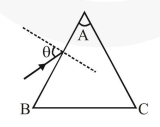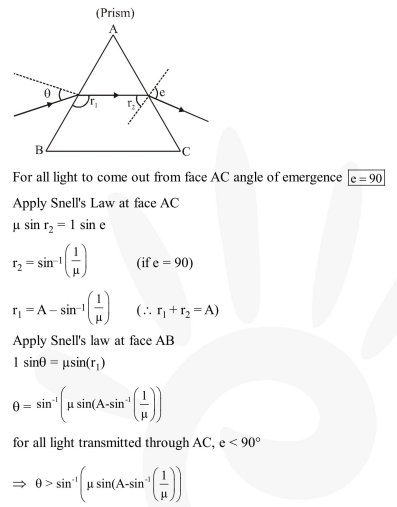# Monochromatic light is incident on a glass prism of angle A.

Question:

Monochromatic light is incident on a glass prism of angle A. If the refractive index of the material of the prism is $\mu$, a ray, incident at an angle $\theta$, on the face $A B$ would get transmitted through the face $\mathrm{AC}$ of the prism provided :1. $\theta>\cos ^{-1}\left[\mu \sin \left(\mathrm{A}+\sin ^{-1}\left(\frac{1}{\mu}\right)\right)\right]$

2. $\theta<\cos ^{-1}\left[\mu \sin \left(\mathrm{A}+\sin ^{-1}\left(\frac{1}{\mu}\right)\right)\right]$

3. $\theta>\sin ^{-1}\left[\mu \sin \left(\mathrm{A}-\sin ^{-1}\left(\frac{1}{\mu}\right)\right)\right]$

4. $\theta<\sin ^{-1}\left[\mu \sin \left(A-\sin ^{-1}\left(\frac{1}{\mu}\right)\right)\right]$

Correct Option: , 3

Solution: